# Generating Reflectance Curves from sRGB Triplets (Page 10)

Update 6/4/2019: I’ve just developed a new method that gives even better natural-reflectance-matching results than the ILLSS method above, with considerably less computational effort required (comparable to that of LLSS). Here it is:

#### Least Hyperbolic Tangent Slope Squared (LHTSS) Method

Recall that the Least Log Slope Squared (LLSS) method generates reflectance curves that are strictly positive, without requiring explicit bounds in the optimization statement. The lower bound is handled implicitly in the logarithmic transformation. This section presents a newly developed approach to generate reflectances that are strictly within the 0-1 range, also not needing explicit bounds. Why is this useful? The methods that use explicit bounds (presented above: ILSS and ILLSS) tend to have abrupt discontinuities in slope when a bounding constraint is engaged. This makes the reflectance curves seem somewhat unnatural. The LHTSS method described in this section makes reflectance curves that gently approach the upper and/or lower bounds, and match the reflectance curves of commercial paints and pigments even better than any of the previous methods above.

The Least Hyperbolic Tangent Slope Squared (LHTSS) method uses a transformation defined by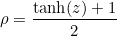to keep all reflectance values between 0 and 1. Below is a plot of the hyperbolic tangent function:

Note how the curve approaches +1/-1 as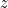grows large in either the positive or negative directions. By adding 1 to tanh and then dividing by 2, the upper and lower bounds shift to 1 and 0. This causes the reflectances to remain strictly between 0 and 1 for any value of:

The least slope optimization statement for the Hyperbolic Tangent Slope Squared method is: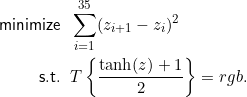Noting that the first derivative of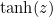is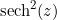, the stationary conditions of the Lagrangian function associated with this optimization comprise a system of 39 nonlinear equations and 39 unknowns: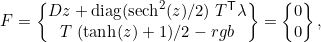where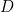is the 36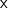36 tridiagonal matrix of finite differencing constants presented earlier.

Newton’s method solves this system of equations with ease, typically in a fairly small number iterations. Noting that the first derivative of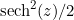is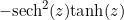, the Jacobian matrix of first partial derivatives of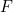is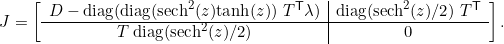The change in the variables with each Newton iteration is found by solving the linear system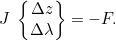At each iteration, the values ofand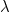are updated using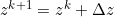and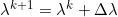.

Here is a Matlab program for the LHTSS (Least Hyperbolic Tangent Slope Squared) method. It also works in the free Octave software. It handles the two cases of sRGB = (0,0,0) and (255,255,255) specially, since the reflectance curves associated with them fall outside the strict interval (0->1) imposed by the hyperbolic tangent transform.

If you prefer not to work in a programming environment, here is an Excel spreadsheet that implements the LHTSS method. Just follow the instructions at the top of the spreadsheet. It uses the “Solver” add-in within Excel, which you may need to load. Here are instructions for doing that.

An example of how this hyperbolic tangent method (LHTSS) compares to the logarithmic method (LLSS) and the regular least slope squared method (LSS), when applied to the Munsell color 7.5R 5/16, is shown in the figure below.Munsell 7.5R 5/16 (purple) compared to LSS (red), LLSS (orange), and LHTSS (blue). (Click to enlarge.)

This figure demonstrates an instance where LSS has negative elements, LLSS has elements >1, and LHTSS corrects both of these deficiencies. It is quite striking how well the LHTSS reconstruction matches the measured reflectance curve of this particular Munsell chip.

To check just how well the curves produced by LHTSS compare to the reflectance curves measured from 1296 Munsell chip colors, the “reflectance match measure, RMM” was computed and added to the previous table:

 Name Max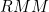MeanLLS, Linear Least Squares 2.46 0.88 LSS, Least Slope Squared 1.11 0.17 ILSS, Iterative Least Slope Squared 1.04 0.16 LLSS, Least Log Slope Squared 0.92 0.15 ILLSS, Iterative Least Log Slope Squared 0.86 0.15 LHTSS, Least Hyperbolic Tangent Slope Squared 0.84 0.14

It is evident that LHTSS performs the best of all methods with regard to the comparison to Munsell reflectance curves.

The computational effort required for the LHTSS method is comparable to that of LLSS. The computational effort study performed above, using 140,608 sRGB triplets (every value possible in intervals of five), showed that the LLSS method required 6.77 iterations on average. The LHTSS method requires an average of 5.66 iterations, so its average run times are somewhat smaller (approx 5%).

________________________________________
Update 1/18/20: These methods (including the new LHTSS method) have been published in a paper called “Numerical Methods for Smoothest Reflectance Reconstruction,” in the journal Color Research and Application (citation: Burns SA. Numerical methods for smoothest reflectance reconstruction. Color Res Appl. 2020;45(1):8-21). Contact me if you’d like a copy of this paper.

________________________________________

#### Acknowledgments

This work has been a tremendous learning experience for me, and I want to thank several people who graciously posted high quality material, upon which most of my work has been based: Bruce Lindbloom, Bruce MacEvoy, Zsolt Kovacs-Vajna, David Briggs, and Paul Centore. If you are interested in learning more about color theory, these five links are excellent places to start!

________________________________________

________________________________________